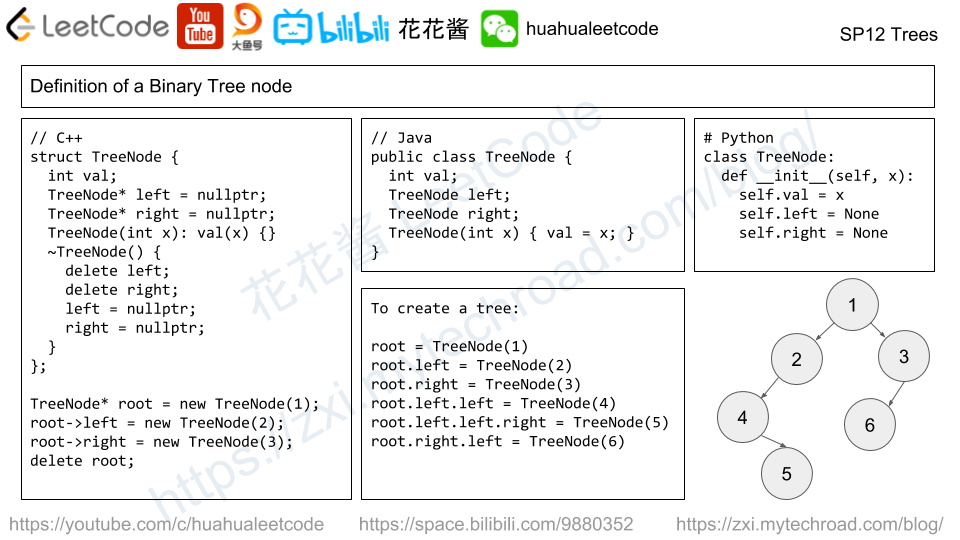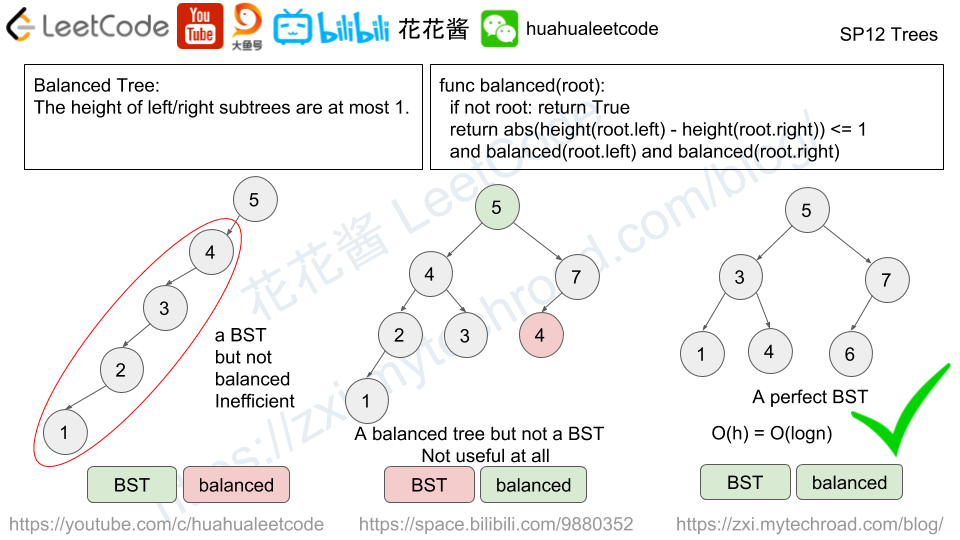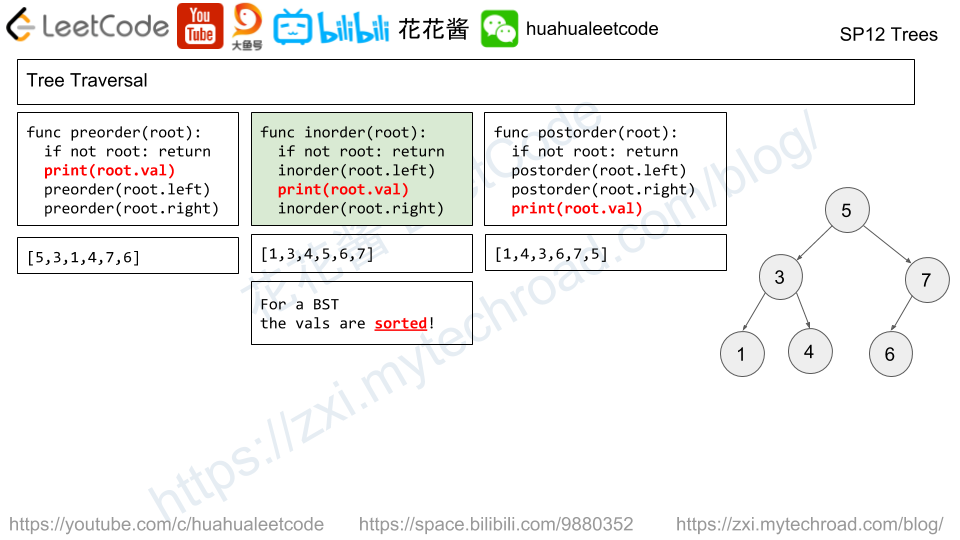# Posts tagged as “BST”

Given a binary search tree, write a function kthSmallest to find the kth smallest element in it.

Note:
You may assume k is always valid, 1 ≤ k ≤ BST’s total elements.

Example 1:

Input: root = [3,1,4,null,2], k = 1
3
/ \
1   4
\
2
Output: 1

Example 2:

Input: root = [5,3,6,2,4,null,null,1], k = 3
5
/ \
3   6
/ \
2   4
/
1
Output: 3


What if the BST is modified (insert/delete operations) often and you need to find the kth smallest frequently? How would you optimize the kthSmallest routine?

Solution: Inorder traversal

Time complexity: O(n)
Space compleixty: O(n)

## C++

Binary tree is one of the most frequently asked question type during interview.

The candidate needs to understand the recursively defined TreeNode and solve the problem through recursion.# Problem

Given the root node of a binary search tree, return the sum of values of all nodes with value between L and R (inclusive).

The binary search tree is guaranteed to have unique values.

Example 1:

Input: root = [10,5,15,3,7,null,18], L = 7, R = 15
Output: 32


Example 2:

Input: root = [10,5,15,3,7,13,18,1,null,6], L = 6, R = 10
Output: 23


Note:

1. The number of nodes in the tree is at most 10000.
2. The final answer is guaranteed to be less than 2^31.

# Solution: In-order traversal

Time complexity: O(n)

Space complexity: O(n)

# Problem

Given an array A, partition it into two (contiguous) subarrays left and right so that:

• Every element in left is less than or equal to every element in right.
• left and right are non-empty.
• left has the smallest possible size.

Return the length of left after such a partitioning.  It is guaranteed that such a partitioning exists.

Example 1:

Input: [5,0,3,8,6]
Output: 3
Explanation: left = [5,0,3], right = [8,6]


Example 2:

Input: [1,1,1,0,6,12]
Output: 4
Explanation: left = [1,1,1,0], right = [6,12]


Note:

1. 2 <= A.length <= 30000
2. 0 <= A[i] <= 10^6
3. It is guaranteed there is at least one way to partition A as described.

# Solution 1: BST

Time complexity: O(nlogn)

Space complexity: O(n)

# Solution 2: Greedy

Time complexity: O(n)

Space complexity: O(1)

# Problem

In an exam room, there are N seats in a single row, numbered 0, 1, 2, ..., N-1.

When a student enters the room, they must sit in the seat that maximizes the distance to the closest person.  If there are multiple such seats, they sit in the seat with the lowest number.  (Also, if no one is in the room, then the student sits at seat number 0.)

Return a class ExamRoom(int N) that exposes two functions: ExamRoom.seat() returning an int representing what seat the student sat in, and ExamRoom.leave(int p) representing that the student in seat number p now leaves the room.  It is guaranteed that any calls to ExamRoom.leave(p) have a student sitting in seat p.

Example 1:

Input: ["ExamRoom","seat","seat","seat","seat","leave","seat"], [,[],[],[],[],,[]]
Output: [null,0,9,4,2,null,5]
Explanation:
ExamRoom(10) -> null
seat() -> 0, no one is in the room, then the student sits at seat number 0.
seat() -> 9, the student sits at the last seat number 9.
seat() -> 4, the student sits at the last seat number 4.
seat() -> 2, the student sits at the last seat number 2.
leave(4) -> null
seat() -> 5, the student​​​​​​​ sits at the last seat number 5.


​​​​Note:

1. 1 <= N <= 10^9
2. ExamRoom.seat() and ExamRoom.leave() will be called at most 10^4 times across all test cases.
3. Calls to ExamRoom.leave(p) are guaranteed to have a student currently sitting in seat number p.

# Solution: BST

Use a BST (ordered set) to track the current seatings.

Time Complexity:

init: O(1)

seat: O(P)

leave: O(logP)

Space complexity: O(P)

Mission News Theme by Compete Themes.